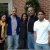Other

#### Description:

The topics covered in Unit 2 include: Integers and Absolute Value, Adding Integers, Subtracting Integers, Multiplying and Dividing Integers, and the Coordinate System.

#### Subjects:

• Mathematics > General
• Education > General

#### Keywords:

Integers and Absolute Value Adding Integers Subtracting Integers Multiplying and Dividing Integers the Coordinate System

English

#### Access Privileges:

Public - Available to anyone

#### Collections:

For Instructors: Project Based Pre-Algebra
Update Standards?

#### MA.6.1.2.a: Mathematics

Fluently divide multi-digit numbers using the standard algorithm.

#### MA.6.1.2.b: Mathematics

Fluently add, subtract, multiply, and divide multi-digit decimals using the standard algorithm for each operation.

#### MA.6.1.3.b.iv: Mathematics

Explain when two ordered pairs differ only by signs, the locations of the points are related by reflections across one or both axes.

#### MA.6.1.3.b.vi: Mathematics

Find and position pairs of integers and other rational numbers on a coordinate plane.

#### MA.6.1.3.d: Mathematics

Solve real-world and mathematical problems by graphing points in all four quadrants of the coordinate plane including the use of coordinates and absolute value to find distances between points with the same first coordinate or the same second coordinate.

#### MA.6.2.1.b.i: Mathematics

Write expressions that record operations with numbers and with letters standing for numbers.

#### MA.6.2.1.b.ii: Mathematics

Identify parts of an expression using mathematical terms (sum, term, product, factor, quotient, coefficient) and describe one or more parts of an expression as a single entity.

#### MA.6.2.1.b.iii: Mathematics

Evaluate expressions at specific values of their variables including expressions that arise from formulas used in real-world problems.

#### MA.6.2.1.b.iv: Mathematics

Perform arithmetic operations, including those involving whole-number exponents, in the conventional order when there are no parentheses to specify a particular order (Order of Operations).

#### MA.6.2.2.c.i: Mathematics

Recognize that a variable can represent an unknown number, or, depending on the purpose at hand, any number in a specified set.

#### MA.6.4.1.c.i: Mathematics

Draw polygons in the coordinate plane given coordinates for the vertices.
Curriki Rating
On a scale of 0 to 3
3
On a scale of 0 to 3

This resource was reviewed using the Curriki Review rubric and received an overall Curriki Review System rating of 3, as of 2013-07-26.

#### Component Ratings:

Technical Completeness: 3
Content Accuracy: 3
Appropriate Pedagogy: 0